# CBSE Class 10 Maths: Explore The Division Algorithm For Polynomial

###### ByRamraj Saini###### Synopsis

The Division Algorithm for Polynomials is a key topic covered in the CBSE Class 10 curriculum. In this article, we will present a complete and easy-to-follow explanation of this concept. You'll get a clear grasp of the steps involved, and we'll use examples to make it even more understandable. With our expert guidance, you can prepare efficiently for your exams.###### Synopsis

The Division Algorithm for Polynomials is a key topic covered in the CBSE Class 10 curriculum. In this article, we will present a complete and easy-to-follow explanation of this concept. You'll get a clear grasp of the steps involved, and we'll use examples to make it even more understandable. With our expert guidance, you can prepare efficiently for your exams.

The CBSE Class 10 Mathematics curriculum is a comprehensive journey through various essential mathematical concepts, each contributing to building a strong foundation for higher studies. Among these concepts, the Division Algorithm for Polynomials is a vital tool for simplifying polynomial division. As you gear up for your exams, this article serves as your ultimate preparation guide to understanding and mastering the Division Algorithm for Polynomials.

## Understanding Polynomials And Polynomial Division

Before we dive into the intricacies of the Division Algorithm, let's establish a solid understanding of the fundamental concepts it relies upon. A polynomial is a mathematical expression constructed from variables and coefficients. It is widely used to represent a variety of real-world situations, such as calculating areas, volumes, and growth patterns.

Population Growth: Polynomials are used to model population growth. The population (P) at a specific time (t) can be approximated using a polynomial equation that involves coefficients and powers of time:
P(t) = antn + a(n-1)t(n-1) + ... + a2t2 + a1t + a

The highest power of variable (t) is n. Therefore, the degree of the polynomial is n.

Economic Revenue: In economics, polynomial equations help predict revenue. The revenue (R) generated from selling a certain quantity (x) of a product can be represented as a polynomial equation with coefficients and powers of x:
R(x) =anxn + a(n-1)x(n-1) + ... + a2x2 + a1x + a

The highest power of variable (x) is n. Therefore, the degree of the polynomial is n.

Polynomials can have terms of different degrees, which are the highest exponents of the variables they contain.

Polynomial division, on the other hand, involves the process of dividing one polynomial by another. The aim is to obtain both a quotient polynomial and a remainder polynomial. The quotient represents how many times the divisor "fits" into the dividend, while the remainder is the leftover part after the division process.

## What Is Division Algorithm?

The Division Algorithm for Polynomials is a systematic procedure that guides us through the process of polynomial division. This algorithm ensures a structured approach to tackling even the most complex polynomial division problems. Here are the steps involved:

Step 1: Arrange Polynomials Begin by arranging both the dividend and the divisor in descending order of their degrees. This step is crucial to ensure a consistent and organised approach.

Step 2: Divide Highest Degree Terms Divide the highest degree term of the dividend by the highest degree term of the divisor. This quotient term becomes the first term of the quotient polynomial.

Step 3: Subtract and Multiply Multiply the entire divisor by the obtained quotient term and subtract the result from the dividend. This step yields a new polynomial, which will be the dividend for the next iteration.

Step 4: Repeat the Process Continue the process by repeating steps 2 and 3 with the new dividend obtained from the previous step. Keep repeating until the degree of the new dividend is less than the degree of the divisor.

Also Read | Class 10: How Can I Get 100 Marks In Maths

## Step-By-Step Examples

To grasp the Division Algorithm more effectively, let's walk through a few step-by-step examples:

Question: Find the quotient of (x3 - 8x2 + 19x - 12) by (x - 1)

Solution:

Put the dividend, (x3 - 8x2 + 19x - 12) inside the long division bar and divisor, (x - 1) outside the division symbol as demonstrated below.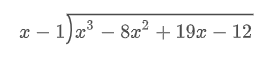Begin by considering the highest exponent, which is x, for divisor and the dividend term x3. Then, figure out a term that, when you multiply it by x, gives you x3. In this case, it's x2.

Multiply the divisor by x2 and write the result below the dividend. This way, similar terms line up neatly, as shown below.Now, consider the next term, which, when multiplied by x, gives you -7x2. In this case, that term is -7x.

Multiply this term, -7x, by the divisor (x - 1), and write down the result as demonstrated below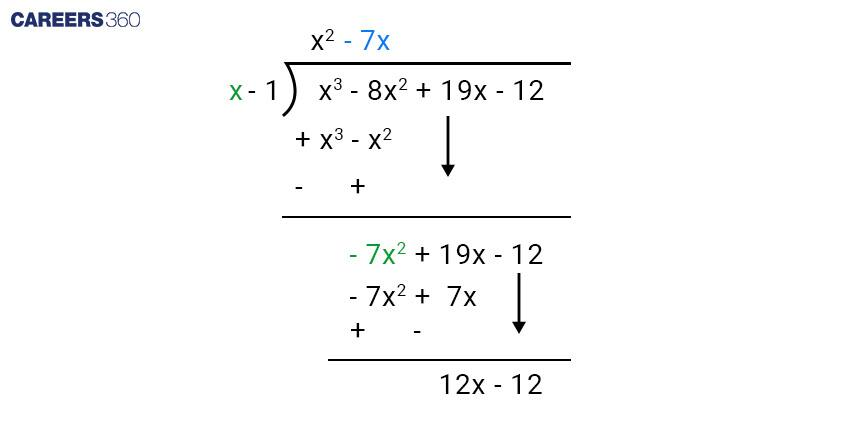Next, find a term that, when multiplied by x, equals 12x. In this case, that term is 12. Write down the result as demonstrated below.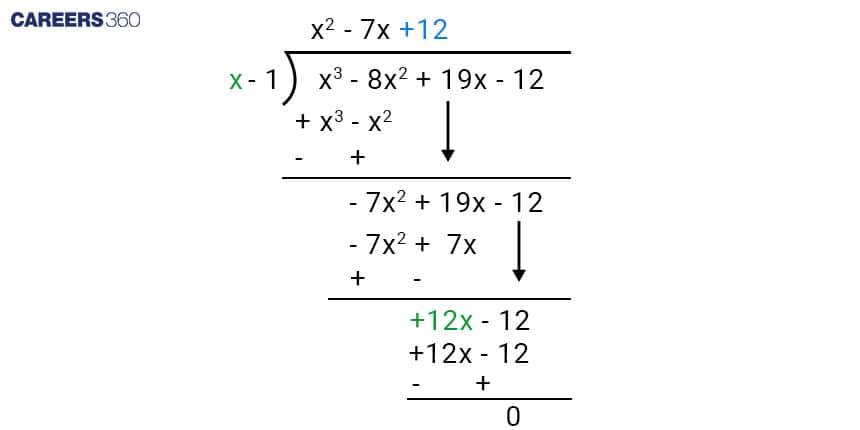It's evident that all terms cancel out, resulting in a remainder of zero. Consequently, the quotient becomes x2 − 7x + 12, and the remainder is zero.

In a broader context, if we have two polynomials, f(x) and g(x), where g(x) is not equal to zero, we can find q(x) and r(x) such that:

Dividend = (Divisor ∗ Quotient) + Remainder

f(x) = g(x)q(x) + r(x)

Where r(x) = 0 or the degree of r(x) is less than the degree of g(x).

This concept is known as the Division Algorithm For Polynomials.

• If r(x) is zero, then g(x) is called factor of f(x).

• If deg f(x) ≥ deg g(x) then deg q(x) = deg f(x) - deg g(x)

## Key Concepts And Tips

Remainder Theorem: The remainder obtained after polynomial division is directly related to the value of the divisor at a specific point.

Factor Theorem: If the divisor is a factor of the dividend, the remainder will be zero.

Choosing Divisor Terms: When dividing by a polynomial, it's essential to select appropriate terms from the divisor to simplify the process.

Simplifying Quotients: Always strive to simplify quotient terms by factoring out common factors.

## Common Mistakes To Avoid

Learning from mistakes is a crucial part of the learning process. Some common errors to watch out for when applying the Division Algorithm include.

• Mis Arranging polynomials before division.

• Miscalculating quotient terms.

• Forgetting to subtract the result of multiplication from the dividend.

• Neglecting to continue the process until the degree of the new dividend is lower than the divisor's degree.

## Practice Exercises

Sharpen your skills with a set of previous year’s questions based practice exercises that cover various scenarios of polynomial division. These exercises will help you become more comfortable with applying the Division Algorithm and reinforce your understanding.

>> Question 1 (CBSE 2020)

What minimum polynomial must be added to 2x3 - 3x2 + 6x + 7 so that the resulting polynomial will be divisible by x2 - 4x + 8?

Solution

Given,

f(x) = 2x3 - 3x2 + 6x + 7

g(x) = x2 - 4x + 8

Find out: Minimum polynomial which is added to f(x) so that resulting polynomial will be divisible by g(x).

Using Division Algorithm for polynomial which is defined as

If we have two polynomials, f(x) and g(x), where g(x) is not equal to zero, we can find q(x) and r(x) such that:

f(x) = g(x)q(x) + r(x)

Divide a polynomial 2x3 - 3x2 + 6x + 7 by x2 - 4x + 8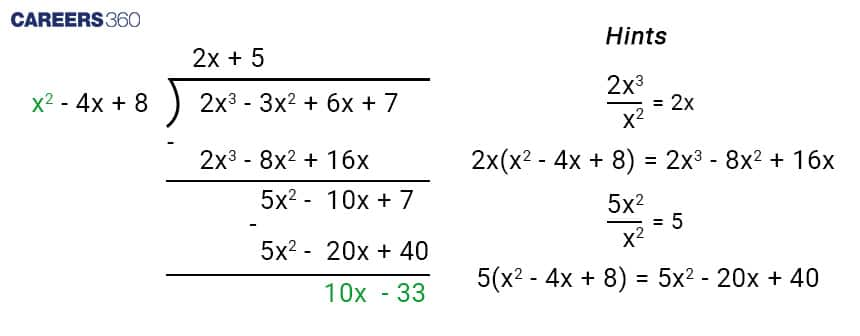Therefore, 2x3 - 3x2 + 6x + 7 = (x2 - 4x + 8) ( 2x + 5 ) + (10x - 33)

Hence, (33 - 10x) should be added to 2x3 - 3x2 + 6x + 7 to make it divisible by x2 - 4x + 8.

>> Question 2 (CBSE 2019)

Apply the division algorithm to check if g(x) = x2 - 3x + 2 is a factor of the polynomial f(x) = x4 - 2x3 - x + 2.

Solution

Given,

To find out → If g(x) is a factor of f(x).

If g(x) is the factor of f(x) then on dividing f(x) by g(x) the remainder should be zero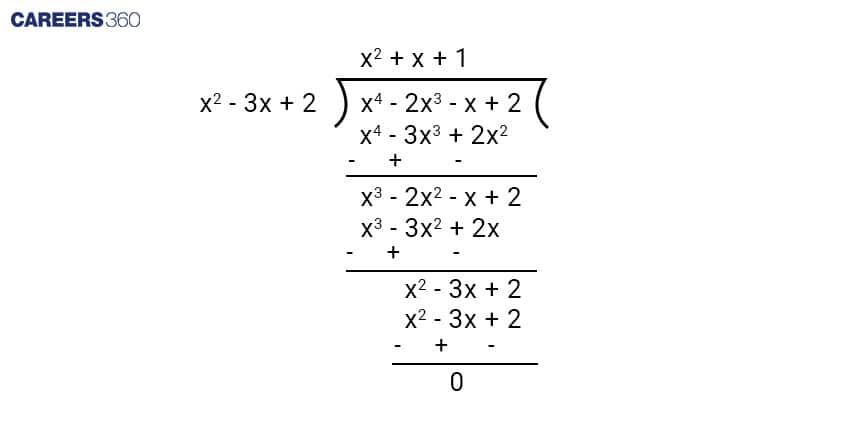As we can see the remainder is zero hence g(x) is a factor of f(x).

## Exam Tips

As you approach your exams, here are some valuable tips to keep in mind:

Time Management: Allocate your time wisely among different questions, considering the complexity of polynomial division problems.

Read Instructions Carefully: Pay close attention to the question requirements—whether you need to find the quotient, remainder, or both.

Check Your Work: Double-check your division calculations to minimise computational errors. Careless mistakes can be avoided with careful review.

## Resources For Further Learning

To deepen your understanding of polynomial division and related concepts, there are various resources available:

Textbooks: Recommended textbooks often provide in-depth explanations, examples, and practice problems.

Online Tutorials: Online tutorials and video lessons can provide visual explanations of complex concepts.

Interactive Tools: Explore interactive tools and software that allow you to experiment with polynomial division scenarios.

Hope you have now gained a comprehensive understanding of the Division Algorithm For Polynomials and the step-by-step application of these principles during the exams.

## Careers360 helping shape your Career for a better tomorrow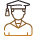#### 250M+

Students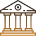#### 30,000+

Colleges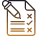#### 500+

Exams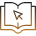E-Books